# CH 34 LESSON 6 GRAPHING QUADRATIC FUNCTIONS IN

• Slides: 16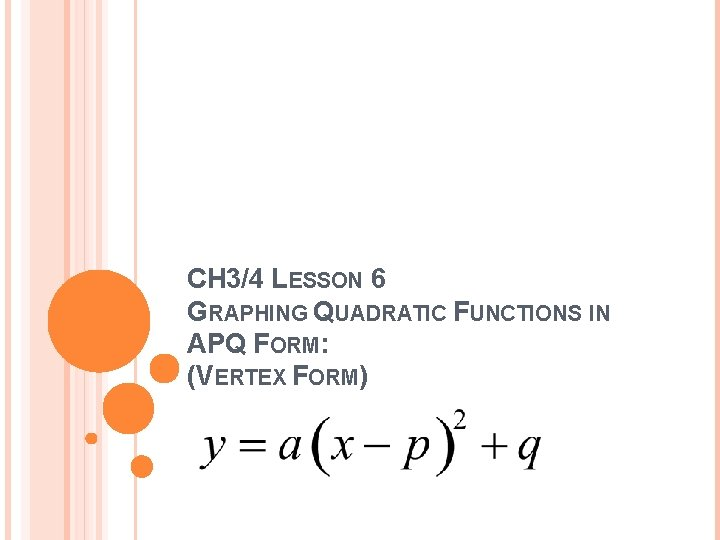CH 3/4 LESSON 6 GRAPHING QUADRATIC FUNCTIONS IN APQ FORM: (VERTEX FORM)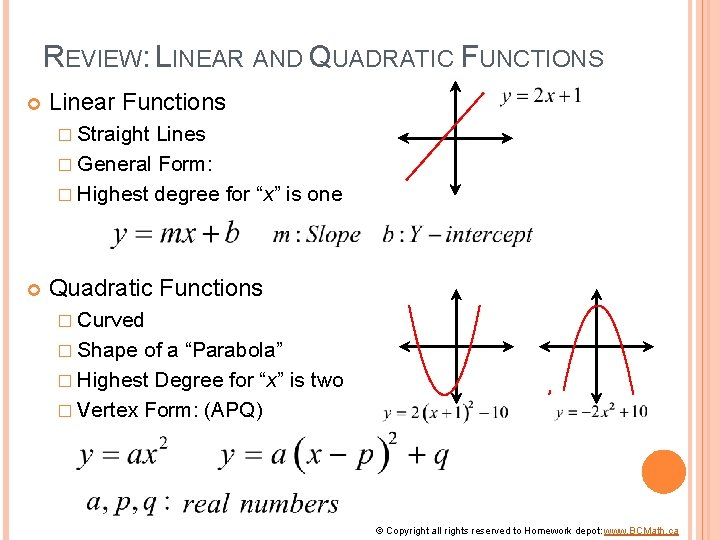REVIEW: LINEAR AND QUADRATIC FUNCTIONS Linear Functions � Straight Lines � General Form: � Highest degree for “x” is one Quadratic Functions � Curved � Shape of a “Parabola” � Highest Degree for “x” is two � Vertex Form: (APQ) © Copyright all rights reserved to Homework depot: www. BCMath. ca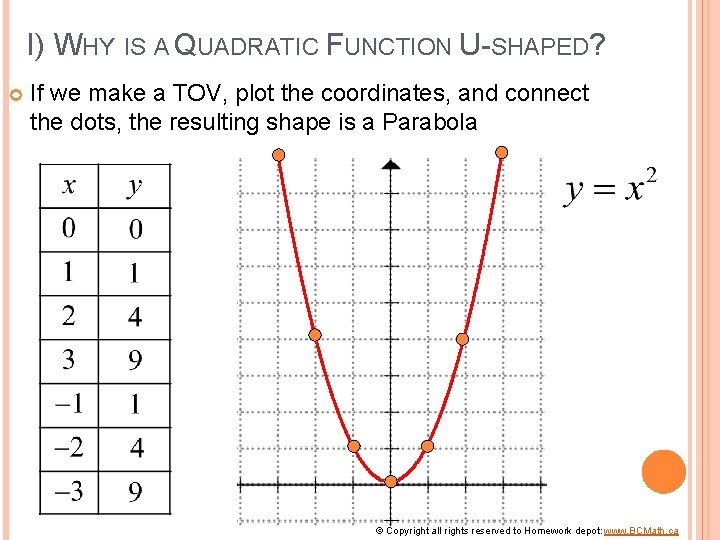I) WHY IS A QUADRATIC FUNCTION U-SHAPED? If we make a TOV, plot the coordinates, and connect the dots, the resulting shape is a Parabola © Copyright all rights reserved to Homework depot: www. BCMath. ca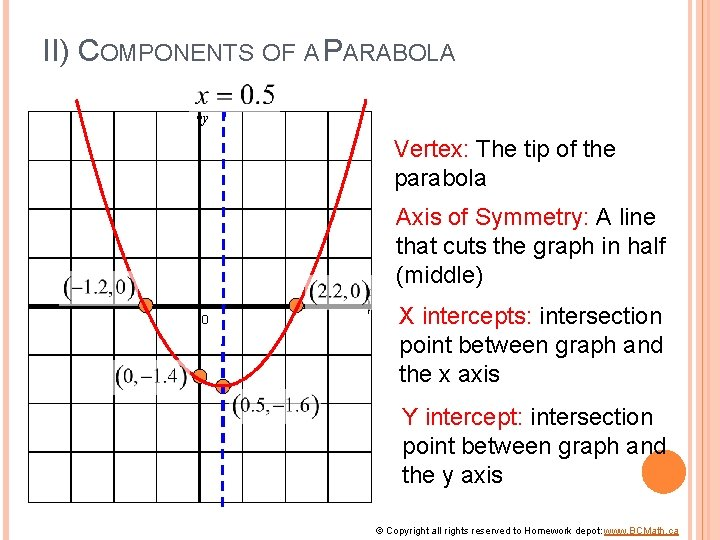II) COMPONENTS OF A PARABOLA y Vertex: The tip of the parabola x 0 Axis of Symmetry: A line that cuts the graph in half (middle) X intercepts: intersection point between graph and the x axis Y intercept: intersection point between graph and the y axis © Copyright all rights reserved to Homework depot: www. BCMath. ca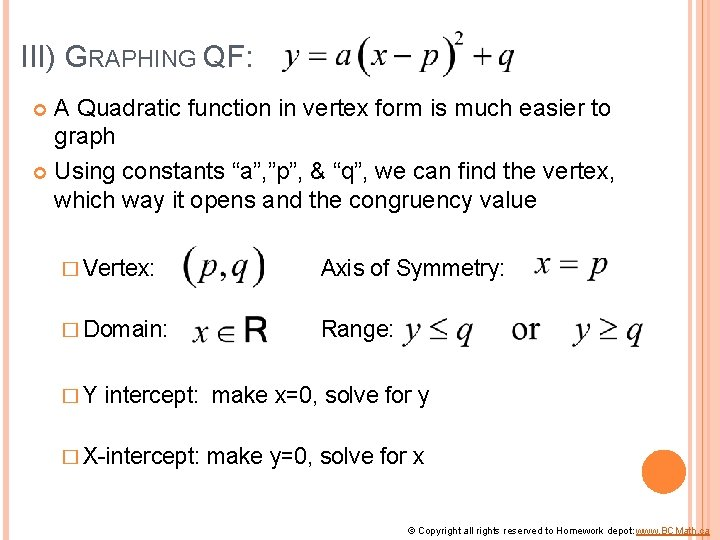III) GRAPHING QF: A Quadratic function in vertex form is much easier to graph Using constants “a”, ”p”, & “q”, we can find the vertex, which way it opens and the congruency value � Vertex: Axis of Symmetry: � Domain: Range: �Y intercept: make x=0, solve for y � X-intercept: make y=0, solve for x © Copyright all rights reserved to Homework depot: www. BCMath. ca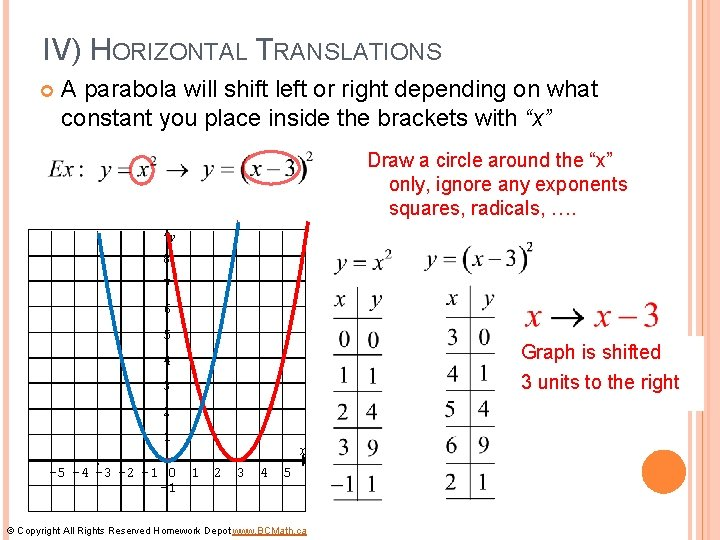IV) HORIZONTAL TRANSLATIONS A parabola will shift left or right depending on what constant you place inside the brackets with “x” Draw a circle around the “x” only, ignore any exponents squares, radicals, …. y 8 7 6 5 Graph is shifted 3 units to the right 4 3 2 1 -5 -4 -3 -2 -1 0 1 -1 x 2 3 4 5 © Copyright All Rights Reserved Homework Depot www. BCMath. caEX: GIVEN THE FOLLOWING EQUATIONS A) GRAPH BOTH EQUATIONS, B) INDICATE WHAT TRANSLATIONS OCCURRED y 8 7 7 6 6 5 5 4 4 3 3 2 2 1 1 x -7 -6 -5 -4 -3 -2 -1 0 1 2 3 -1 Graph is shifted 4 units to the LEFT © Copyright All Rights Reserved Homework Depot www. BCMath. ca xIII) VERTICAL TRANSLATIONS (VT) A Vertical shift (UP or Down) will occur if a constant is added to the equation outside of x 2 The value of “x” is squared first and then we subtract the 3 The constant “ 3” is subtracted outside of the brackets y 8 7 6 5 4 3 2 1 -5 -4 -3 -2 -1 0 -1 x 1 2 3 4 5 -2 -3 -4 © Copyright All Rights Reserved Homework Depot www. BCMath. ca Graph is shifted 3 units DownEX: GIVEN THE FOLLOWING EQUATIONS A) GRAPH BOTH EQUATIONS, B) INDICATE WHAT TRANSLATIONS OCCURRED y 8 7 7 6 6 5 5 4 4 3 3 2 2 1 1 x -5 -4 -3 -2 -1 0 1 2 3 4 5 -1 Graph is shifted 2 units UP © Copyright All Rights Reserved Homework Depot www. BCMath. ca x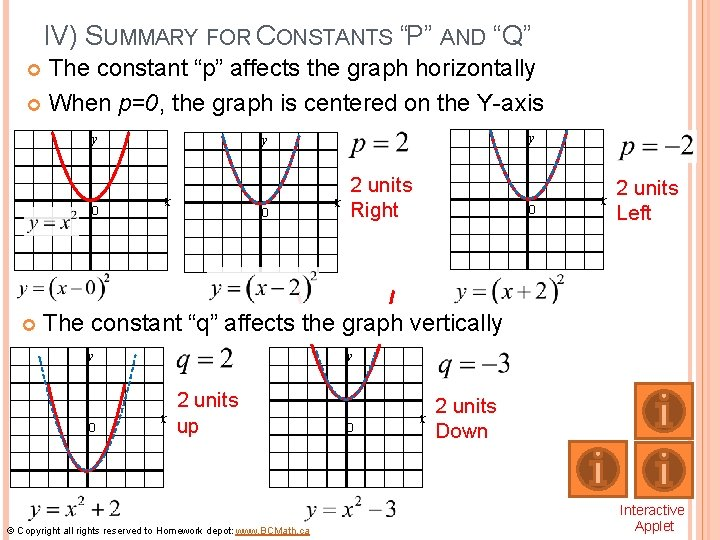IV) SUMMARY FOR CONSTANTS “P” AND “Q” The constant “p” affects the graph horizontally When p=0, the graph is centered on the Y-axis y 0 y y x 0 x 2 units Right 0 x 2 units Left The constant “q” affects the graph vertically y y 0 x 2 units up © Copyright all rights reserved to Homework depot: www. BCMath. ca 0 x 2 units Down Interactive Applet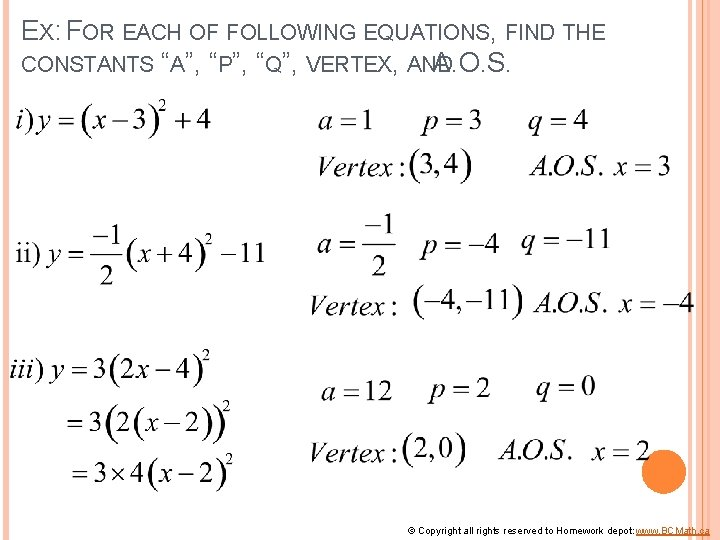EX: FOR EACH OF FOLLOWING EQUATIONS, FIND THE CONSTANTS “A”, “P”, “Q”, VERTEX, AND A. O. S. © Copyright all rights reserved to Homework depot: www. BCMath. ca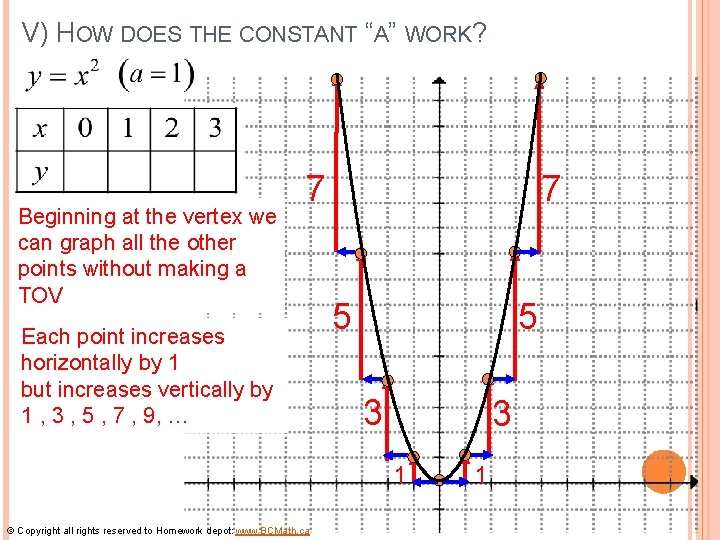V) HOW DOES THE CONSTANT “A” WORK? Beginning at the vertex we can graph all the other points without making a TOV 7 Each point increases horizontally by 1 but increases vertically by 1 , 3 , 5 , 7 , 9, … 7 5 5 3 3 1 © Copyright all rights reserved to Homework depot: www. BCMath. ca 1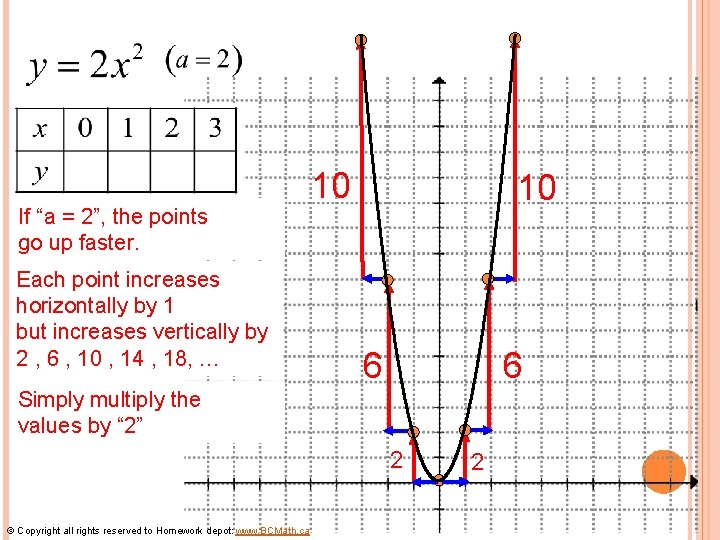If “a = 2”, the points go up faster. Each point increases horizontally by 1 but increases vertically by 2 , 6 , 10 , 14 , 18, … 10 10 6 6 Simply multiply the values by “ 2” 2 © Copyright all rights reserved to Homework depot: www. BCMath. ca 2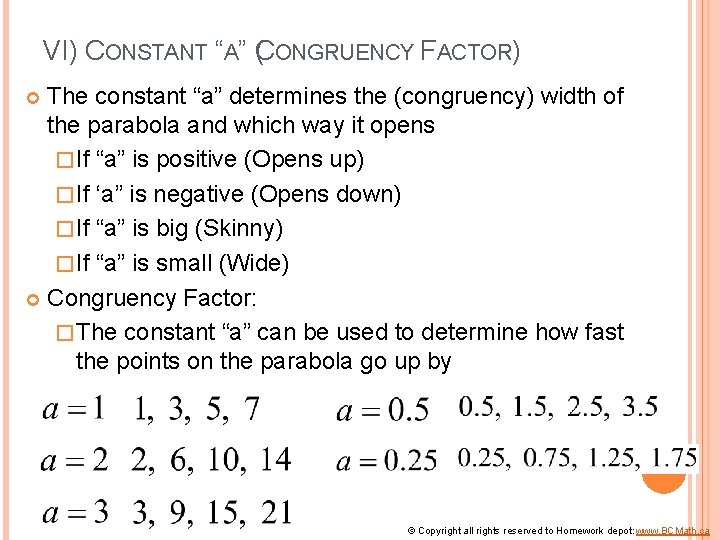VI) CONSTANT “A” (CONGRUENCY FACTOR) The constant “a” determines the (congruency) width of the parabola and which way it opens � If “a” is positive (Opens up) � If ‘a” is negative (Opens down) � If “a” is big (Skinny) � If “a” is small (Wide) Congruency Factor: � The constant “a” can be used to determine how fast the points on the parabola go up by © Copyright all rights reserved to Homework depot: www. BCMath. ca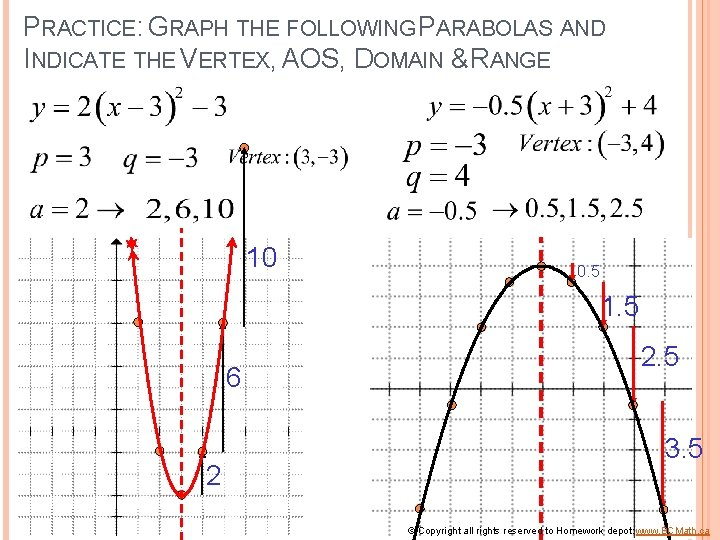PRACTICE: GRAPH THE FOLLOWING PARABOLAS AND INDICATE THE VERTEX, AOS, DOMAIN & RANGE 10 0. 5 1. 5 6 2 2. 5 3. 5 © Copyright all rights reserved to Homework depot: www. BCMath. ca